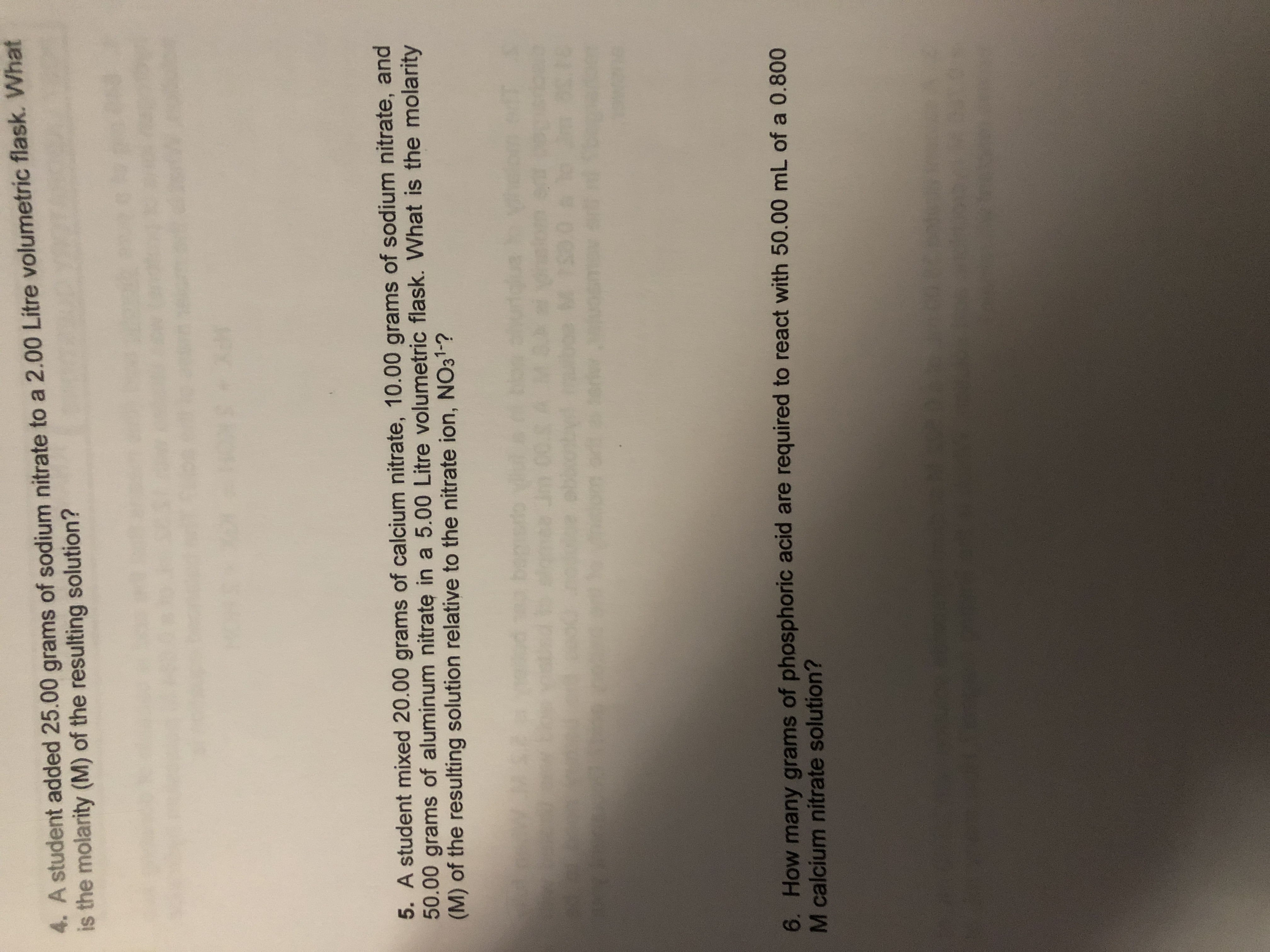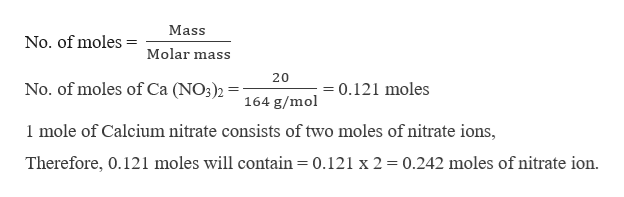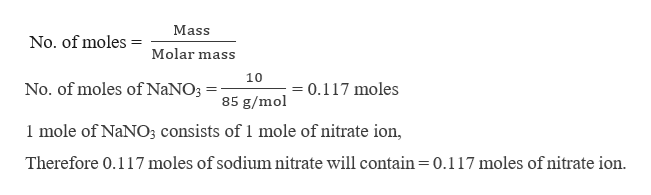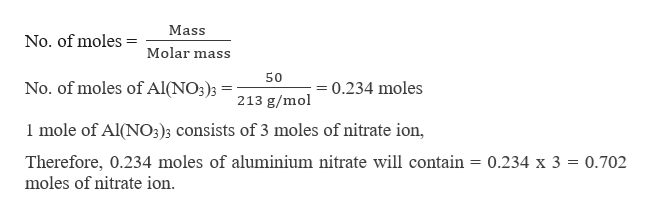# 4. A student added 25.00 grams of sodium nitrate to a 2.00 Litre volumetric flask. Whatis the molarity (M) of the resulting solution?5. A student mixed 20.00 grams of calcium nitrate, 10.00 grams of sodium nitrate, and50.00 grams of aluminum nitrate in a 5.00 Litre volumetric flask. What is the molarity(M) of the resulting solution relative to the nitrate ion, NO31-?6. How many grams of phosphoric acid are required to react with 50.00 mL of a 0.800M calcium nitrate solution?

Question
49 views

Question 5help_outlineImage Transcriptionclose4. A student added 25.00 grams of sodium nitrate to a 2.00 Litre volumetric flask. What is the molarity (M) of the resulting solution? 5. A student mixed 20.00 grams of calcium nitrate, 10.00 grams of sodium nitrate, and 50.00 grams of aluminum nitrate in a 5.00 Litre volumetric flask. What is the molarity (M) of the resulting solution relative to the nitrate ion, NO31-? 6. How many grams of phosphoric acid are required to react with 50.00 mL of a 0.800 M calcium nitrate solution? fullscreen
check_circle

Step 1

The molarity of nitrate ion can be calculated by first calculating the moles of nitrate present in the solution.

Calculating the number of moles of the calcium nitrate in 20 grams.help_outlineImage TranscriptioncloseMass No. of moles Molar mass 20 No. of moles of Ca (NO3)2 = 0.121 moles 164 g/mol 1 mole of Calcium nitrate consists of two moles of nitrate ions, Therefore, 0.121 moles will contain = 0.121 x 2 = 0.242 moles of nitrate ion. fullscreen
Step 2

Now the number of moles of sodium nitrate can be calculated as,help_outlineImage TranscriptioncloseMass No. of moles = Molar mass 10 No. of moles of N2NO3 0.117 moles 85 g/mol 1 mole of NaNO3 consists of 1 mole of nitrate ion, Therefore 0.117 moles of sodium nitrate will contain = 0.117 moles of nitrate ion. fullscreen
Step 3

The number of moles of aluminium nitrate (Al(NO3)...help_outlineImage TranscriptioncloseMass No. of moles = Molar mass 50 No. of moles of Al(NO3); 0.234 moles 213 g/mol 1 mole of Al(NO3)3 consists of 3 moles of nitrate ion, Therefore, 0.234 moles of aluminium nitrate will contain = 0.234 x 3 moles of nitrate ion 0.702 fullscreen

### Want to see the full answer?

See Solution

#### Want to see this answer and more?

Solutions are written by subject experts who are available 24/7. Questions are typically answered within 1 hour.*

See Solution
*Response times may vary by subject and question.
Tagged in

### General Chemistry